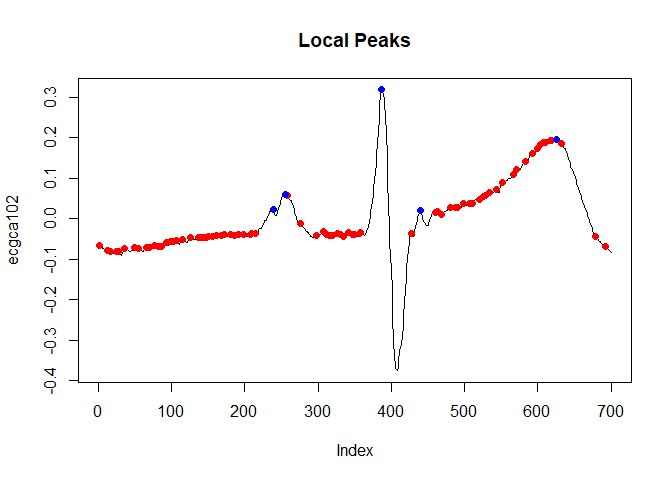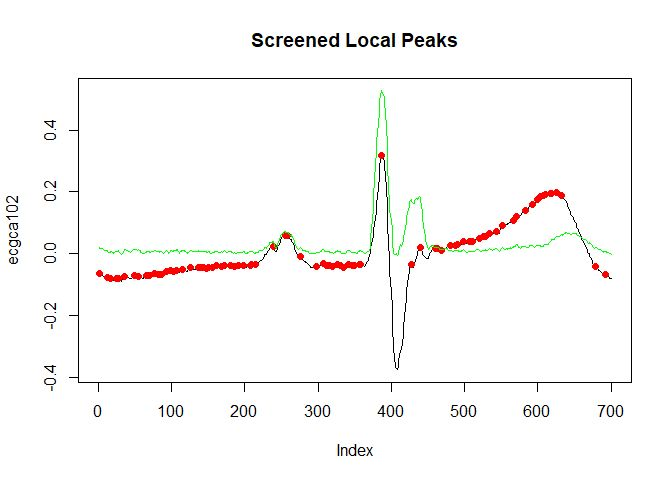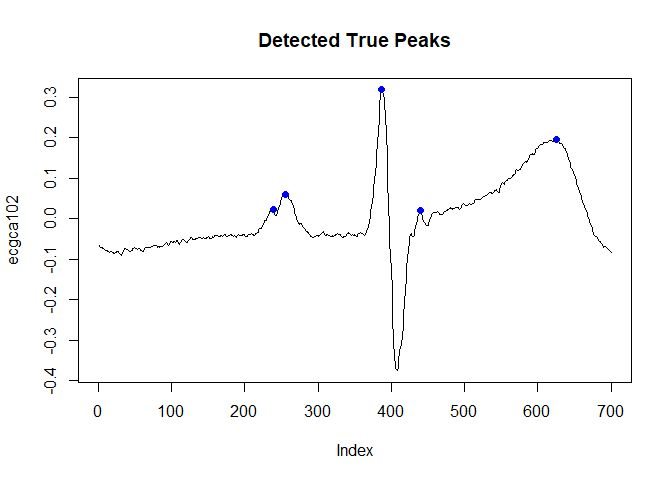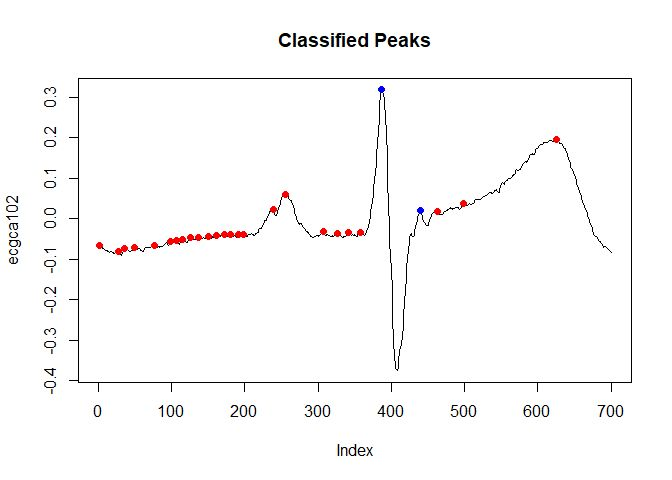# Introduction to scorepeak

#### 2019-08-20

There are true peaks and false peaks (noise) in time series data.

We need to formalize the notion of a peak to distinguish true peaks from false peaks.

An approach is to define a peak function, which gives a score of a data point.

The score indicates how high the possibility that the data point is a true peak is.

We can classify a peak as true or false by the score.

scorepeak package provides several peak functions and its building blocks.

## Mathematical Notation

$$T=x_1,x_2,\cdots,x_n$$: an univariate uniformly sampled time series data

$$x_i$$: $$i^{th}$$ point in T

$$n$$: length of time series data

$$l$$: window size ($$l\geq3$$ and $$l$$ is odd)

$$k$$: half of window size ($$k=\frac{l-1}{2}$$)

$$N^r(k,i,T)=<x_{i+1},x_{i+2},\cdots,x_{i+k}>$$: the sequence of right temporal neighbors

$$N^l(k,i,T)=<x_{i-k},x_{i-k+1},\cdots,x_{i-1}>$$: the sequence of left temporal neighbors

$$N(k,i,T)=N^r(k,i,T){\cdot}N^l(k,i,T)$$: the sequence of left and right temporal neighbors ($$\cdot$$ denotes concatenation)

$$N'(k,i,T)=N^r(k,i,T){\cdot}\{x_i\}{\cdot}N^l(k,i,T)$$: the sequence of the data point and its left and right temporal neighbors

$$max(A)$$: maximum in a set $$A$$

$$min(A)$$: minimum in a set $$A$$

$$mean(A)$$: average of elements in a set $$A$$

$$s(A)$$: standard deviation of elements in a set $$A$$

## Peak Functions

### Type 1

Type 1 of peak function $$S_1$$ computes the average of (i) the maximum among the signed distances of $$x_i$$ from its right neighbors and (ii) the maximum among the signed distance of $$x_i$$ from its left neighbors.

$$\large S_1(k,i,T)=\dfrac{max_{j{\in}N^l(k,i,T)}(x_i-x_j)+max_{j{\in}N^r(k,i,T)}(x_i-x_j)}{2}$$

### Type 2

Type 2 of peak function $$S_2$$ computes the signed distance of the $$x_i$$ from the average of its left and right temporal neighbors.

$$\large S_2(k,i,T)=x_i-mean(N(k,i,T))$$

### Type 3

Type 3 of peak function $$S_3$$ computes the product of the signed distance of the $$x_i$$ from the larger one of the average of $$N^l(k,iT)$$ and the average of $$N^r(k,iT)$$ and the standard deviation of $$N'(k,iT)$$.

$$\large S_3(k,i,T)=(x_i-max(mean(N^l(k,i,T)), mean(N^r(k,i,T))))*s(N'(k,i,T))$$

## Example of Use

See the data shown below. We can see many local peaks in the data.

The points indicate the local peaks.

library(scorepeak)
data("ecgca102")
local_peaks <- detect_localmaxima(ecgca102)
idx_true_peaks <- c(239, 255, 387, 439, 625)
plot(ecgca102, type = "l", main = "Local Peaks")
points(which(local_peaks), ecgca102[local_peaks], col = "red", pch = 19)
points(idx_true_peaks, ecgca102[idx_true_peaks], col = "blue", pch = 19)Which local peaks are true peaks?

It depends on your purpose.

Here, assume that the blue points are true peaks and the red points indicate false peaks.

Then, let’s detect the true peaks.

First, screen the local peaks.

Second, apply a peak function (score_type1 function).

local_peaks_screened <- detect_localmaxima(ecgca102, 13)
score <- score_type1(ecgca102, 51)
plot(ecgca102, type = "l", main = "Screened Local Peaks", ylim = c(-0.38, 0.53))
points(which(local_peaks), ecgca102[local_peaks], col = "red", pch = 19)
points(seq(length(score)), score, type = "l", col = "green")Finally, binarize the screened local peaks by a threshold.

true_peaks <- score > 0.03 & local_peaks_screened
plot(ecgca102, type = "l", main = "Detected True Peaks")
points(which(true_peaks), ecgca102[true_peaks], col = "blue", pch = 19)Although we binarized the local peaks by the user-defined threshold value in the above,

we can determine threshold value automatically by machine learning if we prepare a train set.

By the way, we can combine peak function with clustering algorithm.

classified_peaks <- cluster::pam(score, 2, cluster.only = TRUE)
cp1 <- classified_peaks == 1 & local_peaks_screened
cp2 <- classified_peaks == 2 & local_peaks_screened
plot(ecgca102, type = "l", main = "Classified Peaks")
points(which(cp1), ecgca102[cp1], col = "red", pch = 19)
points(which(cp2), ecgca102[cp2], col = "blue", pch = 19)## Boundary Condition

The above definitions of the peak functions are valid if $$i$$ is greater than $$k$$ and smaller than $$n-k$$.

However, the definitions are not valid if $$i$$ is smaller than or equal to $$k$$ and greater than or equal to $$n-k$$.

We need to consider how to treat the boundary ($$k>=i,i>=n-k$$).

The peak functions in scorepeak package have boundary argument, which determines how to treat boundary.

The valid values of boundary are shown below.

• “reflecting”, “r”: Reflecting Boundary Condition

• “periodic”, “p”: Periodic Boundary Condition

### Reflecting Boundary Condition

Extend data reflectively as follows.

$$T=x_n,\cdots,x_2,x_1,x_2,\cdots,x_n,x_{n-1},\cdots,x_1$$

### Periodic Boundary Condition

Extend data periodically as follows.

$$T=x_1,\cdots,x_n,x_1,x_2,\cdots,x_n,x_1,\cdots,x_n$$

Consider only data points that is not boundary ($$k<i<n-k$$).

## Building Blocks of Peak Functions

The peak functions shown above are useful.

However, you may need other peak function.

You can build your peak function out of building blocks shown below.

• max_neighbors: computes maximum of temporal neighbors

• min_neighbors: computes minimum of temporal neighbors

• mean_neighbors: computes mean of temporal neighbors

• sd_neighbors: computes standard deviation of temporal neighbors

The above functions have side argument.

side determines which temporal neighbors will be used in calculation.

The valid values of side are shown below.

• “right”, “r”: right temporal neighbors ($$N^r(k,i,T)$$)

• “left”, “l”: : left temporal neighbors ($$N^l(k,i,T)$$)

• “both”, “b”: left and right temporal neighbors ($$N(k,i,T)$$)

• “all”, “a”: the data point and its left and right temporal neighbors ($$N'(k,i,T)$$)